<img height="1" width="1" alt="" style="display:none" src="https://www.facebook.com/tr?id=940171109376247&amp;ev=PageView&amp;noscript=1">

# Dedicated to Teachers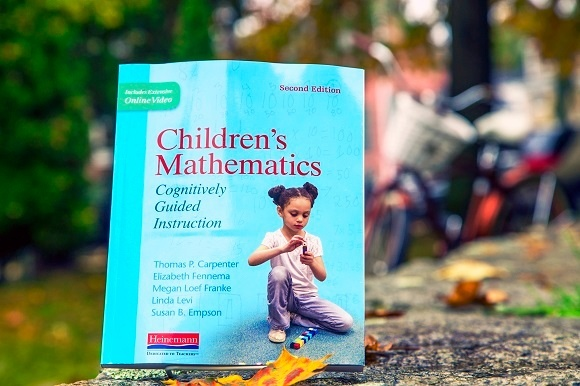Children’s Mathematics, Extending Children’s Mathematics, and Thinking Mathematically are essential reading for teachers who want to understand how children learn mathematics. Grounded in the Cognitively Guided Instruction (CGI) philosophy pioneered by the authors, these books have helped hundreds of thousands of teachers learn more about children’s intuitive mathematical thinking and teach math more confidently. In today's blog, co-author Linda Levi talks about the connections between the three books in the CGI family.

by Linda Levi

Teachers often ask me which of the three CGI books they should read first or read next. Choosing which CGI book to read ultimately depends on what you are interested in. I write this hoping to help you understand the focus of each of the CGI books and how each book relates the others.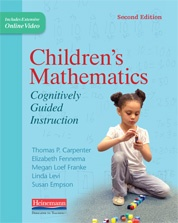Tom Carpenter, Elizabeth Fennema, Megan Franke, Susan Empson and I wrote Children’s Mathematics: Cognitively Guided Instruction to help teachers understand how primary-aged children think about mathematics and how instruction can be guided by children’s mathematical thinking. Over 30 years of research on young children’s thinking in the areas of addition, subtraction, multiplication, division and place value resulted in exceptionally robust frameworks describing children’s mathematical development. Virtually all children progress through the same trajectory when learning these concepts. No matter what curriculum you use, children are going to think about addition, subtraction, multiplication, and division in the ways described in these research frameworks. Children’s Mathematics helps teachers understand these frameworks without having to read 30 years of research!

Teachers who worked with the ideas in Children’s Mathematics quickly came to the conclusion that instruction can be guided by students’ thinking even when teaching mathematical concepts not addressed in our book. These teachers found the research-based frameworks in Children’s Mathematics to be exceptionally helpful and they wanted to learn about frameworks describing students’ thinking in other areas. We have been asked to write CGI books for geometry, fractions, integers, statistics, probability, formal algebra, and even Calculus!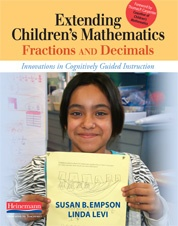Susan Empson and I wrote Extending Children’s Mathematics: Fractions and Decimals to help teachers understand research frameworks on how students think about fractions and decimals and how fraction and decimal instruction can be guided by students’ thinking. Extending Children’s Mathematics supports teachers’ fraction and decimal instruction much in the same way that Children’s Mathematics supports teachers’ whole number instruction.

In traditional instruction, fractions are often treated as a brand new topic unrelated to anything students have learned before. The standard algorithms for fraction computation indeed look quite different than the standard algorithms for whole number computation. In contrast, Extending Children’s Mathematics shows how fractions can be a natural extension of what children already understand about whole numbers. For example, a student who can solve a problem about 4 people sharing 12 brownies can use the concepts they already understand about whole number division to solve a problem about 4 people sharing 3 brownies. This previous understanding is key to helping them develop an understanding of ¾. Students can learn to add, subtraction, multiply and divide fractions based on what they already know about computation with whole numbers. Fractions should not be a brand new topic—the properties of operations work the same for fractions as they do for whole numbers. Teachers can capitalize on these similarities to help students learn fractions with understanding.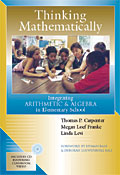Several teachers have asked me when we are going to write a CGI book about properties of operations. The good news is that we already wrote it! Tom Carpenter, Megan Franke and I wrote Thinking Mathematically: Integrating Arithmetic and Algebra in Elementary School to help teachers engage their students in the process of generating, representing, discussing and justifying general principles of arithmetic. The properties of operations are the central principles of arithmetic and are discussed in depth in this book.

Thinking Mathematically is useful for all teachers who are working to integrate more algebraic thinking into their math instruction. In Thinking Mathematically, algebra is not viewed as a separate topic but rather as a topic students can engage in when they are solving typical computation problems—either word problems or equations. Integrating algebra and arithmetic enhances the learning of arithmetic at the same time as it provides students with a foundation for learning formal algebra later on.

Thinking Mathematically differs somewhat from our other books; although we still present frameworks describing students’ thinking, we also present frameworks describing mathematical concepts and processes. The mathematical concepts we describe include the properties of operations and equations as other general principles regarding how operations and equations work. The processes that we describe focus on the different stages in developing an understanding of general principles in mathematics.

All three of our books are based on the premise that all students can learn math with understanding and that learning is enhanced when teachers base instruction on students’ mathematical thinking. I hope these books are useful to you and that this helps you understand how one book relates to the others.

♦ ♦ ♦ ♦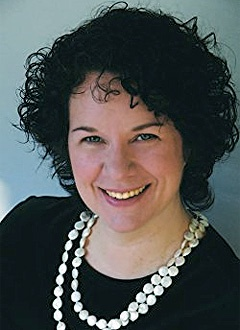Linda Levi is Director of Professional Development in Cognitively Guided Instruction for Teachers Development Group, a nonprofit organization dedicated to increasing all students' understanding and achievement through teacher professional development. She currently works with schools, districts, education cooperatives and State Departments of Education to provide CGI professional development.